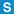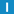# class Clock

Library: Foundation
Package: DateTime

## Description

A Clock stores a monotonic* clock value with (theoretical) microseconds resolution. Clocks can be compared with each other and simple arithmetic is supported.

[*] Note that Clock values are only monotonic if the operating system provides a monotonic clock. The monotonic() function can be used to check whether the system's clock is monotonic.

Monotonic Clock is available on Windows, Linux, OS X and on POSIX platforms supporting clock_gettime() with CLOCK_MONOTONIC.

Clock values are relative to a system-dependent epoch time (usually the system's startup time) and have no relation to the time of day.

## Types

### ClockDiff

typedef Int64 ClockDiff;

Difference between two ClockVal values in microseconds.

### ClockVal

typedef Int64 ClockVal;

Monotonic clock value in microsecond resolution.

## Constructors

### Clock

Clock();

Creates a Clock with the current system clock value.

### Clock

Clock(
ClockVal tv
);

Creates a Clock from the given clock value.

### Clock

Clock(
const Clock & other
);

Copy constructor.

## Destructor

### ~Clock

~Clock();

Destroys the Clock.

## Member Functions

### accuracystatic ClockDiff accuracy();

Returns the system's clock accuracy in microseconds.

### elapsedClockDiff elapsed() const;

Returns the time elapsed since the time denoted by the Clock instance. Equivalent to Clock() - *this.

### isElapsedbool isElapsed(
ClockDiff interval
) const;

Returns true if and only if the given interval has passed since the time denoted by the Clock instance.

### microsecondsClockVal microseconds() const;

Returns the clock value expressed in microseconds since the system-specific epoch time (usually system startup).

### monotonicstatic bool monotonic();

Returns true if and only if the system's clock is monotonic.

### operator !=bool operator != (
const Clock & ts
) const;

### operator +Clock operator + (
ClockDiff d
) const;

### operator +=Clock & operator += (
ClockDiff d
);

### operator -Clock operator - (
ClockDiff d
) const;

### operator -

ClockDiff operator - (
const Clock & ts
) const;

### operator -=Clock & operator -= (
ClockDiff d
);

### operator <bool operator < (
const Clock & ts
) const;

### operator <=bool operator <= (
const Clock & ts
) const;

### operator =

Clock & operator = (
const Clock & other
);

### operator =

Clock & operator = (
ClockVal tv
);

### operator ==bool operator == (
const Clock & ts
) const;

### operator >bool operator > (
const Clock & ts
) const;

### operator >=bool operator >= (
const Clock & ts
) const;

### rawClockVal raw() const;

Returns the clock value expressed in microseconds since the system-specific epoch time (usually system startup).

Same as microseconds().

### resolutionstatic ClockDiff resolution();

Returns the resolution in units per second. Since the Clock class has microsecond resolution, the returned value is always 1000000.

### swap

void swap(
Clock & clock
);

Swaps the Clock with another one.

### update

void update();

Updates the Clock with the current system clock.

## Variables

### CLOCKVAL_MAXstatic const ClockVal CLOCKVAL_MAX;

Maximum clock value.

### CLOCKVAL_MINstatic const ClockVal CLOCKVAL_MIN;

Minimum clock value.